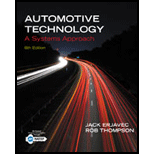Chapter 15, Problem 10RQAutomotive Technology: A Systems A...

6th Edition
Jack Erjavec + 1 other
ISBN: 9781133612315

Solutions

Chapter
SectionAutomotive Technology: A Systems A...

6th Edition
Jack Erjavec + 1 other
ISBN: 9781133612315
Textbook Problem

Which type of resistor is commonly used in automotive circuits? Fixed value SteppedVariableAll of the above

To determine

The type of resistor commonly used in an automotive circuit.

Explanation

A resistor is a device used to limit the flow of current in an electrical circuit. In an automotive circuit, the commonly used resistors are:

1. Fixed value
2. Stepped or tapped, and
3. Variable

As the name suggests, a fixed value is a type of resistor whose electrical resistance value is fixed and does not change in the circuit. These resistor decrease the amount of voltage applied to a component...

Still sussing out bartleby?

Check out a sample textbook solution.

See a sample solution

The Solution to Your Study Problems

Bartleby provides explanations to thousands of textbook problems written by our experts, many with advanced degrees!

Get Started

For Problems 16.20 through 16.23, use the cutting planes shown to draw the sectional views.

Engineering Fundamentals: An Introduction to Engineering (MindTap Course List)

Given that P=120lb and Q=130lb, find the rectangular representation of P+Q.

International Edition---engineering Mechanics: Statics, 4th Edition

5. Describe a data dictionary and list the types of information it contains.

Systems Analysis and Design (Shelly Cashman Series) (MindTap Course List)